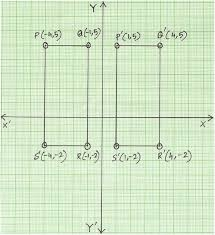# Circumference 9211

The rectangle has a circumference of 30 cm, and its width is 3 cm shorter than its length. What is its area?

a =  6 cm
b =  9 cm
S =  54 cm2

### Step-by-step explanation:

a=b-3
2(a+b)=30

a=b-3
2•(a+b)=30

a-b = -3
2a+2b = 30

a = 6
b = 9

Our linear equations calculator calculates it.Did you find an error or inaccuracy? Feel free to write us. Thank you!

Tips for related online calculators
Do you have a linear equation or system of equations and looking for its solution? Or do you have a quadratic equation?
Do you want to convert length units?

#### Grade of the word problem:

We encourage you to watch this tutorial video on this math problem: